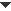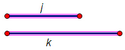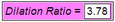﻿ Menus > Transform Menu > Mark Ratio/Mark Segment Ratio/Mark Scale Factor

# Mark Ratio/Mark Segment Ratio/Mark Scale FactorSelection prerequisites: Any number of objects including two segments, one value without units, or three collinear points

This Transform menu command has three forms: Mark Segment Ratio, Mark Scale Factor, and Mark Ratio.

The command marks the most recently selected ratio or scale factor as the ratio for future dilations. The form of the command depends on your selections:

 Select: For this command: To use this ratio or scale factor: Two segments (j, k) Mark Segment Ratio j / k One value with no units Mark Scale Factor The selected value Three collinear points (A, B, C) Mark Ratio AC / AB

Dilations can use either a fixed ratio (such as ½) or a marked ratio (such as AC / AB). When you dilate by ½, the ratio of dilation never changes; but when you dilate by AC / AB, the dilation changes as the positions of points A, B, and C change.

Use a marked ratio or scale factor when you want your dilated image to change as the marked item changes.Mark Segment Ratio (Two Segments)Selection prerequisites: Any number of objects including two segments 1.Select a segment whose length will be used as the numerator of your ratio.2.Select a second segment whose length will be used as the denominator.3.Choose Transform | Mark Segment Ratio.You will see a brief animation confirming that the ratio has been marked. If the first segment is shorter than the second, the ratio is less than 1 and the dilation will shrink objects toward the marked center. If the first segment is longer than the second, the ratio is greater than 1 and the dilation will stretch objects away from the marked center. You can also mark a segment ratio after choosing With the Dilate dialog box open, click the two segments in your sketch, one after the other.Mark Scale Factor (A Value with No Units)Selection prerequisites: Any number of objects including an undimensioned value (a measurement, parameter, or calculation) 1.Select an undimensioned value  (a pure value, with no units such as inches or degrees)  to be the scale factor.2.Choose Transform | Mark Scale Factor.The selection will flash briefly to confirm that your value has been marked. A convenient way to create a scale factor is to construct a point on a straight object and measure the point’s value. If the magnitude of the value you marked as a scale factor is less than 1, the dilation will shrink objects toward the marked center. If the value’s magnitude is greater than 1, the dilation will expand objects away from the marked center. If the scale factor is less than 0, image objects will be dilated “through the center,” appearing on the opposite side of the center as their pre-images. You can also mark a scale factor after choosing With the Dilate dialog box open, click an undimensioned value in your sketch.Mark Ratio (Three Collinear Points)Selection prerequisites: Any number of objects including three collinear points 1.Select three collinear points in your sketch. The points must be in a straight line.2.Choose Transform | Mark Ratio.You will see a brief animation confirming that the ratio has been marked. When you mark three collinear points, A, B, and C, as a ratio, Sketchpad dilates by the ratio of signed distances AC / AB. If B is on the same side of A as C, the signed ratio of distances is positive; if B is on the opposite side of A as C, the ratio is negative. You may want to use the Measure | Ratio command to display this ratio numerically. One handy way to remember the roles of the three selected points is to think of them as determining a ratio that, if the first selected point was the marked center, would dilate the second selected point to the location of the third selected point. Another way to remember the roles of the points is to think of them forming a number line, with point A representing the origin, B the unit point, and C the point whose value is used as the ratio.